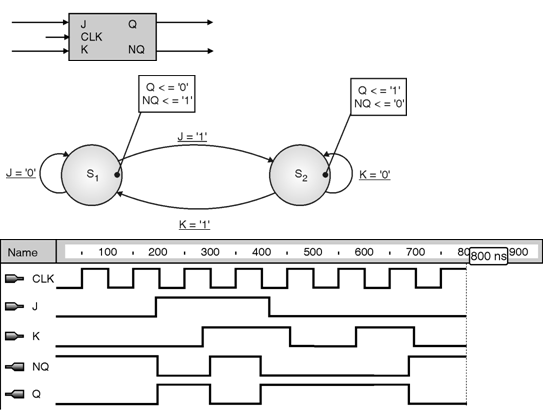Home > finite state machines > Metastability > JK flipflop State Machine

# JK flipflop State Machine

An example of a simple state machine is a J-K flip-flop. A J-K flip-flop is sequential device with two inputs, two outputs, and clock input. The J-K flip-flop outputs change on negative edges of the CLK signal. The NQ output is the inverted Q output.

• The Q output turns '1' when J = '1' and K = '0'.
• The Q output turns '0' when J = '0' and K = '1'.
• The Q output turns the opposite value when both J = '1' and K = '1'.
• The Q output remains unchanged when both J = '0' and K = '0'.
The above description is sufficient to create a state diagram for the J-K flip-flop. Figure below, state diagram includes all basic diagram items, which are :
• States
• Transitions
• Transition condition
• Actions